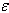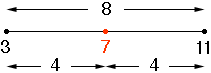SEARCH HOMEMath Central Quandaries & QueriesQuestion from Timothy, a student: 1. Write the interval in absolute value notation i) xE[0,9] ii) xE[-2,20]Hi Timothy,

Let me try a different example, x[3, 11]

The absolute value notation for an interval is anchored at the middle of the interval. The middle of this interval is (11 + 3)/2 = 14/2 = 7. The length of the interval is 11 - 3 = 8 units. The interval then stretches from 4 units to the left of the middle to 4 units to the right of the middle.Thus x is in the interval if the distance between x and 7 is less than or equal to 4.

The distance between x and 7 is |x - 7| and hence the interval can be written

|x - 7| ≤ 4

Let's try another one, x[-5, 8]

The middle of the interval is (8 + (-5))/2 = 3/2
The length of the interval is (8 - (-5)) = 13 so half the length is 13/2

Thus the interval is

|x - 3/2| ≤ 13/2

PennyMath Central is supported by the University of Regina and The Pacific Institute for the Mathematical Sciences.